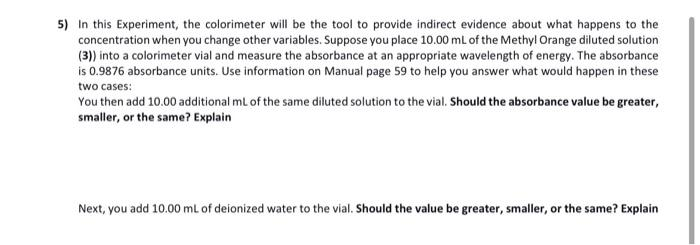# (Solved): 5) In this Experiment, the colorimeter will be the tool to provide indirect evidence about what hap ...5) In this Experiment, the colorimeter will be the tool to provide indirect evidence about what happens to the concentration when you change other variables. Suppose you place $$10.00 \mathrm{~mL}$$ of the Methyl Orange diluted solution (3)) into a colorimeter vial and measure the absorbance at an appropriate wavelength of energy. The absorbance is $$0.9876$$ absorbance units. Use information on Manual page 59 to help you answer what would happen in these two cases: You then add $$10.00$$ additional $$\mathrm{mL}$$ of the same diluted solution to the vial. Should the absorbance value be greater, smaller, or the same? Explain Next, you add $$10.00 \mathrm{~mL}$$ of deionized water to the vial. Should the value be greater, smaller, or the same? Explain

We have an Answer from Expert